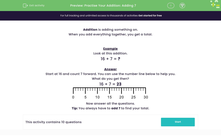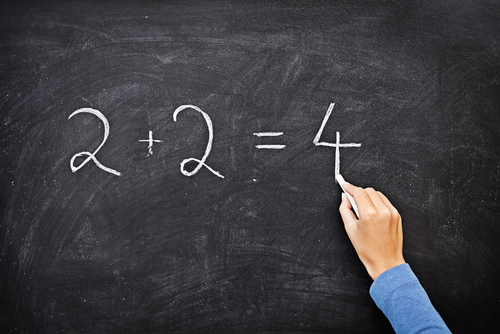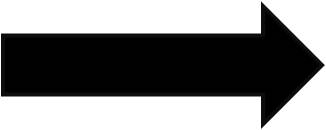### Comprehensive & curriculum aligned

In this worksheet, students will add the numbers 7, 8 or 9 to complete a calculation.Key stage:  KS 1

Curriculum topic:   Number: Addition and Subtraction

Curriculum subtopic:   Add and Subtract to 20

Difficulty level:#### Worksheet Overview

When you add everything together, you get a total.Example

16 + 7 = ?

Start at 16 and count 7 forwards.

What do you get then?

16 + 7 = 23If you use the number line to help you, start on the number 16 and bounce your finger along the line 7 places to the right. You should get to 23.

Remember that when you add, you always move to the right.Let's try adding 8 to a number now.

19 + 8 = ?

You need to count forwards 8 places. If you use the number line, start at 19 and bounce your finger along 8 places to the right and what do you reach?

19 + 8 = 2715 + 9 = ?

You need to move forwards 9 places on the number line and you'll arrive at 24.

15 + 9 = 24

There is another way that you could work this one out. 9 is one less than 10. If you add on 10 and then subtract 1, it will be the same as adding 9. Does that make sense?

Let's try: 15 + 10 = 25 - 1 = 24

It works!!Let's try some questions now.

### What is EdPlace?

We're your National Curriculum aligned online education content provider helping each child succeed in English, maths and science from year 1 to GCSE. With an EdPlace account you’ll be able to track and measure progress, helping each child achieve their best. We build confidence and attainment by personalising each child’s learning at a level that suits them.

Get started